### How to Calculate Yield in Excel

People who invest in bonds are always anxious to know the current yield, the yield to maturity and the yield to call of the bonds they purchased. Using Excel, you can develop a bond yield calculator easily with the help of a number of formulas. You just need to enter the inputs like face value, coupon rate, years to maturity etc and Excel will calculate the bond yield and display it for you.

### Calculate Yield

Open Excel and save your file as yield.xlsx. Type “Face Value” in A1, “Annual Coupon Rate” in A2, “Annual Required Return” in A3, “Years to Maturity” in A4, “Years to Call” in A5, “Call Premium” in A6, “Payment Frequency” in A7, “Value of Bond” in A9, “Current Yield” in A11, “Yield to Maturity” in A12 and “Yield to Call” in A13. You can format these cells and make them bold. Now your screen will look like this:Select cells B1 and B9. Right click and go to Format Cells.You will get a window like this:From the Category: section on the left hand side, select Currency. Select \$ English (U.S.) from the Symbol: drop down on the right hand side. Now your screen will look like this: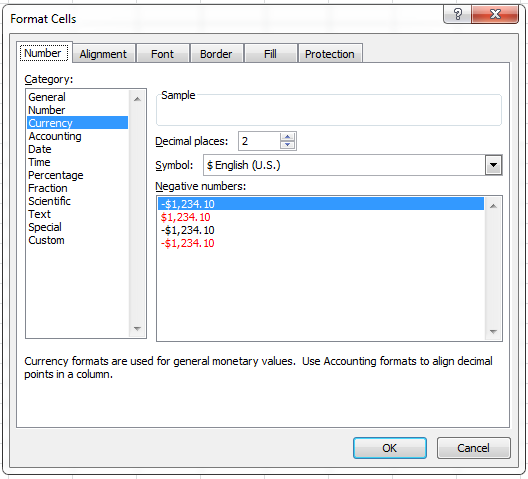Click OK.

Select cells B2, B3, B6, B11, B12 and B13. Right click and go to Format Cells. You will get a new window. From the Category: section on the left hand side, select Percentage. Now your screen will look like this: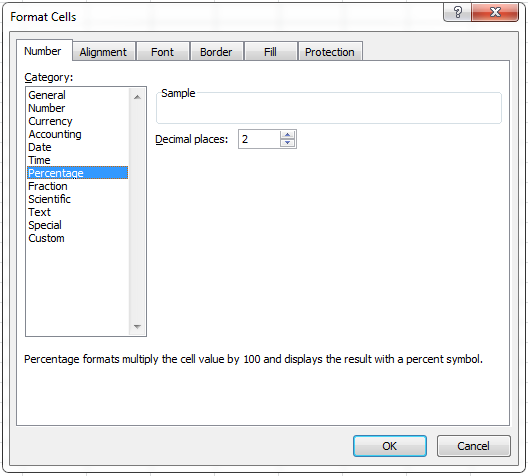Click OK.

Enter some reasonable values in the cells B1, B2, B3, B4 and B7.To calculate the present value of the bond, click the cell B9. Go to Formulas (main menu) –> Financial (in the Function Library group) and select the PV function.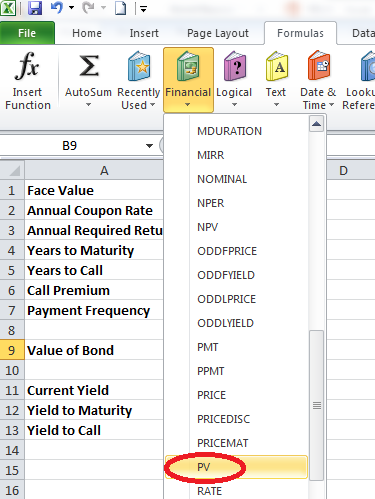You will get a window like this: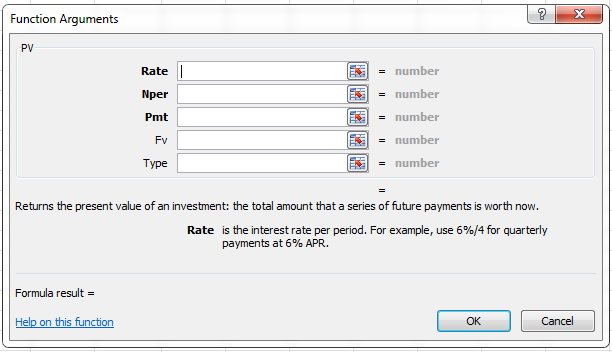In the Rate, Nper, Pmt and Fv textboxes, enter the values B3/B7, B4*B7, B2/B7*B1 and B1 respectively. Now your window like this:Click OK. Now you will get a negative value in the cell B9. Now go to the formula bar and add a – sign just after the = sign like this: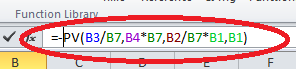To calculate the current yield, click inside the cell B11 and enter the formula “=(B1*B2)/B9” (without double quotes).

To calculate the yield to maturity, click inside the cell B12. Go to Formulas (main menu) –> Financial (in the Function Library group) and select the RATE function. You will get a window like this:In the Rate, Nper, Pmt and Fv textboxes, enter the values B4*B7, B2*B1/B7, -B9 and B1 respectively. Now your window will look like this: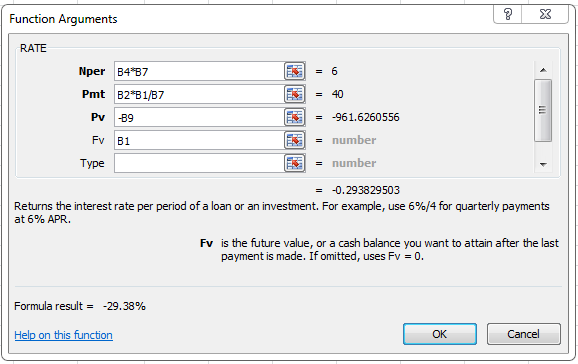Click OK. As this value is for the half year, go to the formula bar and add *B7 at the end of the formula like this: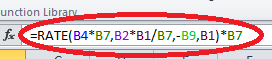Enter reasonable values in the cells B5 and B6 (say 1 and 3).

To calculate the yield to call, click inside the cell B13. Go to Formulas (main menu) –> Financial (in the Function Library group) and select the RATE function. You will get a new window. In the Rate, Nper, Pmt and Fv textboxes, enter the values B5*B7, B2*B1/B7, -B9 and B1*(1+B6) respectively. Now your window will look like this:Click OK. Go to the formula bar and add *B7 at the end of the formula like this: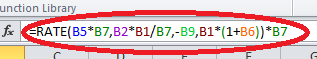Now your bond yield calculator will look like this: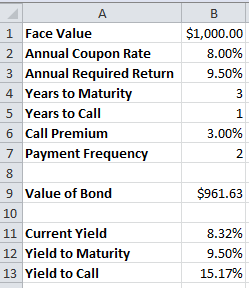By submitting the face value, coupon rate, required return, years to maturity, years to call, call premium and payment frequency, you get the current yield, yield to maturity and yield to call with this bond yield calculator. You can try changing the inputs and observe the difference in the output.

## Template

```Further reading: